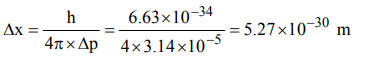## Atomic Structure Questions and Answers Part-9

1. The uncertainty in the position of an electron $\left(mass=9.1 × 10^{-28}g\right)$    moving with a velocity of $3.0 × 10^{4}cms^{-1}$   accurate upto 0.011% will be
a) 1.92 cm
b) 7.68 cm
c) 0.175 cm
d) 3.84 cm.

Explanation:2. The uncertainty in the momentum of an electron is $1.0 × 10^{-5}kg ms^{-1}$  . The uncertainty in its position will be $\left(h = 6.62 × 10^{-34}kg m^{2}s^{-1}\right)$
a) $1.05 × 10^{-26}m$
b) $1.05 × 10^{-28}m$
c) $5.27 × 10^{-30}m$
d) $5.25 × 10^{-28}m$

Explanation:3. Azimuthal quantum number determines the
a) size
b) spin
c) orientation
d) angular momentum of orbitals

Explanation:4. When the value of azimuthal quantum number, l = 2, value of ‘n’ will be
a) 3
b) 4
c) 5
d) any one of these

Explanation: For l = 2 (d subshell) n $\geq$ 3

5. The values of quantum numbers n, l and m for the 5th electron of Boron will be
a) n = 1, l = 0, m = –1
b) n = 2, l = 1, m = –1
c) n = 2, l = 2, m = –1
d) n = 1, l = 2, m = –1

Explanation:6. The total number of orbitals in a shell with principal quantum number ‘n’ is
a) 2n
b) $2n^{2}$
c) $n^{2}$
d) n

Explanation: Number of orbitals with principal quantum number n = n2

7. Which one of the following set of quantum numbers is not possible for 4p electron?
a) $n = 4, l = 1, m = –1,m_{s}=+\frac{1}{2}$
b) $n = 4, l = 1, m = 0,m_{s}=+\frac{1}{2}$
c) $n = 4, l = 1, m = 2,m_{s}=+\frac{1}{2}$
d) $n = 4, l = 1, m = –1,m_{s}=-\frac{1}{2}$

Explanation: For 4p electron n = 4, l = 1, m = –1, 0 + 1 and s = +½ or –½

8. The maximum number of electrons in subshell with l = 2 and n = 3 is
a) 2
b) 6
c) 12
d) 10

Explanation: l = 2 for d subshell hence 10 electrons

9. An electron has principal quantum number 3. The number of its (i) sub-shells and (ii) orbitals would be respectively
a) 3 and 5
b) 3 and 7
c) 3 and 9
d) 2 and 5

10. An $\overline{e}$  has magnetic quantum number as –3, what is its principal quantum number